# RD Sharma Solutions - Chapter 15 - Understanding Shapes-I (Polygons), Class 8, Maths Notes | Study RD Sharma Solutions for Class 8 Mathematics - Class 8

## Class 8: RD Sharma Solutions - Chapter 15 - Understanding Shapes-I (Polygons), Class 8, Maths Notes | Study RD Sharma Solutions for Class 8 Mathematics - Class 8

The document RD Sharma Solutions - Chapter 15 - Understanding Shapes-I (Polygons), Class 8, Maths Notes | Study RD Sharma Solutions for Class 8 Mathematics - Class 8 is a part of the Class 8 Course RD Sharma Solutions for Class 8 Mathematics.
All you need of Class 8 at this link: Class 8

PAGE NO 15.5:

Question 1:

Draw rough diagrams to illustrate the following:
(i) Open curve
(ii) Closed curve

(i) Open curve: A curve in which the beginning and the end points does not cut each other or are different.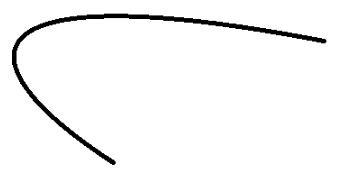(ii) Closed curve: A curve in which the beginning and the end points are the same and cuts each other.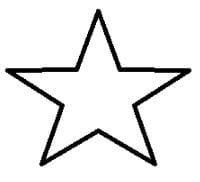Question 2:

Classify the following curves as open or closed: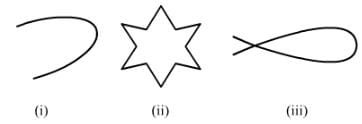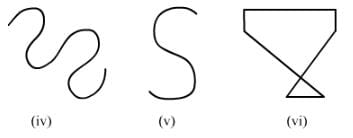Open curve: A curve in which the beginning and end points are different or do not cut each other.
Closed curve: A curve in which the beginning and end points are the same and cut each other.
By the above definitions, we can classify the given figures as follows:
(i) Open curve
(ii) Closed curve
(iii) Closed curve
(iv) Open curve
(v) Open curve
(vi) Closed curve

Question 3:

Draw a polygon and shade its interior. Also draw its diagonals, if any.

In polygon ABCD, AC and BD are the diagonals.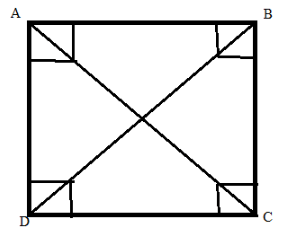PAGE NO 15.6:

Question 4:

Illustrate, if possible, each one of the following with a rough diagram:
(i) A closed curve that is not a polygon.
(ii) An open curve made up entirely of line segments.
(iii) A polygon with two sides.

(i) Polygons are made up of straight lines, not curves.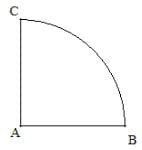(ii) An open curve made up entirely of line segments: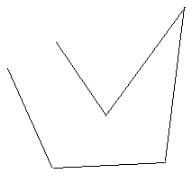(iii) Not possible because polygons are closed figures.

Question 5:

Following are some figures: Classify each of these fugures on the basis of the following:
(i) Simple curve
(ii) Simple closed curve
(iii) Polygon
(iv) Convex polygon
(v) Concave polygon
(vi) Not a curve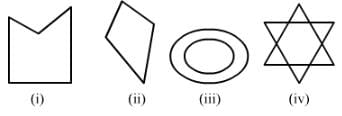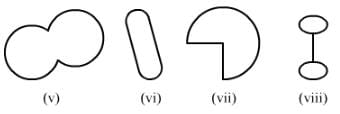(i) It is a simple closed curve and a concave polygon.
(ii) It is a simple closed curve and a convex polygon.
(iii) It is not a curve; hence, it is not a polygon.
(iv) It is not a curve; hence, it is not a polygon.
(v) It is a simple closed curve but not a polygon.
(vi) It is a simple closed curve but not a polygon.
(vii) It is a simple closed curve but not a polygon.
(viii) It is a simple closed curve but not a polygon.

Question 6:

How many diagonals does each of the following have?
(ii) A regular hexagon
(iii) A triangle

An n-sided convex polygon has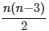diagonals.
(i) A quadrilateral has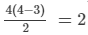diagonals.
There are 2 diagonals in the convex quadrilateral.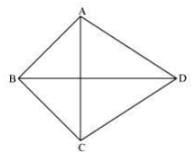(ii) A regular hexagon has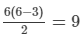diagonals.
There are 9 diagonals in a regular hexagon.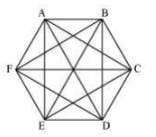(iii) A triangle does not have any diagonal in it.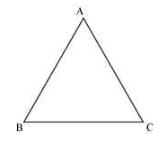Question 7:

What is a regular polygon? State the name of a regular polygon of
(i) 3 sides
(ii) 4 sides
(iii) 6 sides

A polygon that has equal sides and equal angles is called a regular polygon.

(i) Equilateral triangle: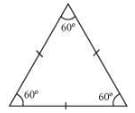(ii) Square: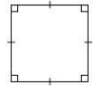(iii) Regular hexagon: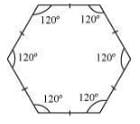The document RD Sharma Solutions - Chapter 15 - Understanding Shapes-I (Polygons), Class 8, Maths Notes | Study RD Sharma Solutions for Class 8 Mathematics - Class 8 is a part of the Class 8 Course RD Sharma Solutions for Class 8 Mathematics.
All you need of Class 8 at this link: Class 8Use Code STAYHOME200 and get INR 200 additional OFF

## RD Sharma Solutions for Class 8 Mathematics

88 docs

Track your progress, build streaks, highlight & save important lessons and more!

,

,

,

,

,

,

,

,

,

,

,

,

,

,

,

,

,

,

,

,

,

,

,

,

,

,

,

;